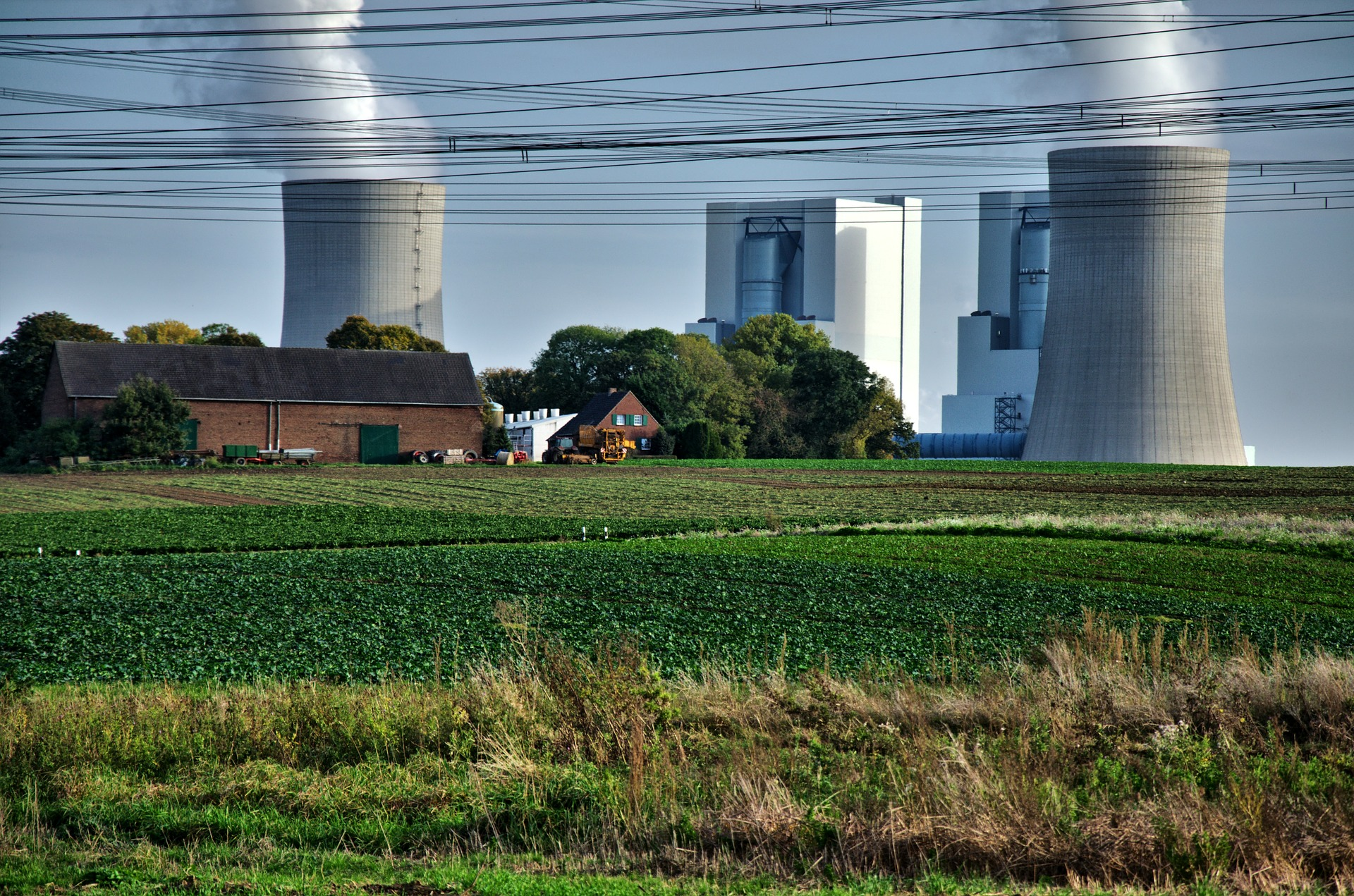## Cooling Tower Makeup Water Calculation with Example

It’s been a while since the last time I write an article about utility system. In this post I want to share you how to calculate cooling tower makeup water.

Cooling tower must be made up because the water level decrease by the time during operation. There are aspect that contribute to calculation of cooling tower makeup water. Those are:

• Evaporation loss
• Drift loss
• Blow down

Total cooling water makeup water requirement = evaporation loss + drift loss + blow down.

Let’s take a look one by one how to calculate each component.

### Evaporation Loss

Evaporation loss is loss of water due to evaporation. It is calculated by this equation:

Evaporation loss = 0.00085 Wc (T1-T2)